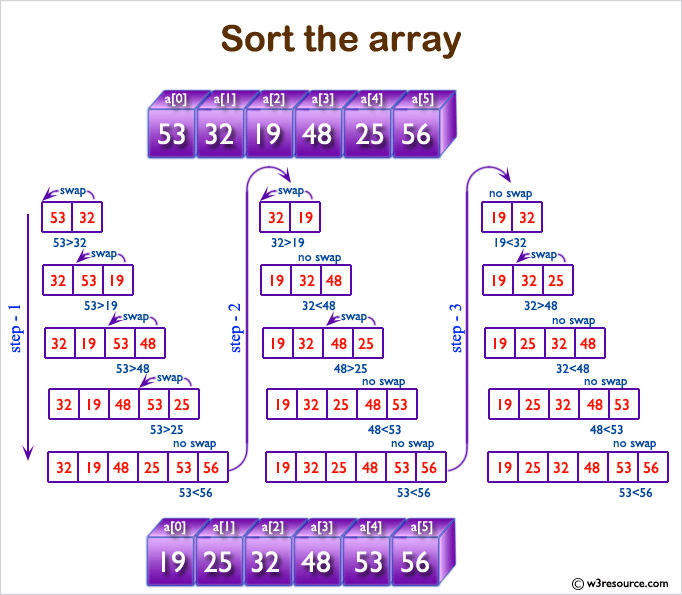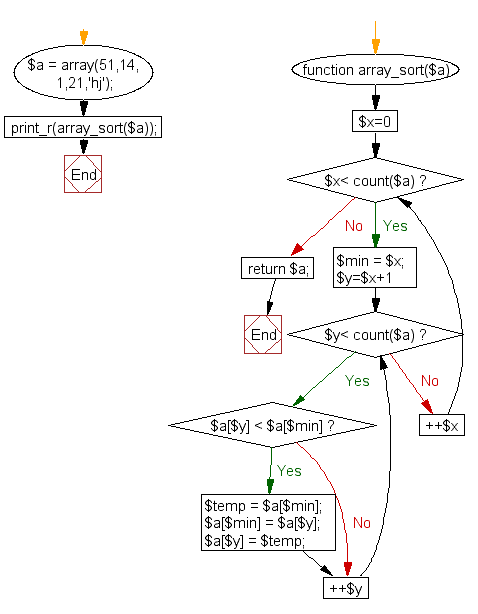﻿ PHP Function Exercise: Sort an array - w3resource# PHP function Exercises: Sort an array

## PHP function: Exercise-4 with Solution

Write a function to sort an array.

Pictorial Presentation:Sample Salution:

PHP Code:

``````<?php
function array_sort(\$a)
{
for(\$x=0;\$x< count(\$a);++\$x)
{
\$min = \$x;
for(\$y=\$x+1;\$y< count(\$a);++\$y)
{
if(\$a[\$y] < \$a[\$min] )
{
\$temp = \$a[\$min];
\$a[\$min] = \$a[\$y];
\$a[\$y] = \$temp;
}
}
}
return \$a;
}
\$a = array(51,14,1,21,'hj');
print_r(array_sort(\$a));
?>
```
```

Sample Output:

```Array
(
 => hj
 => 1
 => 14
 => 21
 => 51
)
```

Flowchart :PHP Code Editor:

Have another way to solve this solution? Contribute your code (and comments) through Disqus.

What is the difficulty level of this exercise?

Test your Programming skills with w3resource's quiz.

﻿

## PHP: Tips of the Day

Formatting a number with leading zeros in PHP

Use sprintf :

`sprintf('%08d', 1234567);`

Alternatively you can also use str_pad:

`str_pad(\$value, 8, '0', STR_PAD_LEFT);`

Ref : https://bit.ly/3fS2OLd